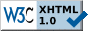# Optimization Lab for Communication and Signal Processing Using MATLAB (OptiLab)

This Lab is about learning how to recognize, model and solve optimization problems. Covered topics include but are not limited to:
• Linear Programming
• Quadratic Programming and Least-Squares Problems
• Regularized Approximation and Estimation
• Second-order Cone Programming
• Semidefinite Programming
• Methodologies to solve a convex problem
• Theoretical analysis
• Duality theory, Karush-Kuhn-Tucker (KKT) conditions of optimality
• Numerical approach
• CVX: Matlab Toolbox for convex optimization problems
• Numerical solvers for convex problems: e.g., SeDuMi, SDPT3
• Application to a wide range of topics, e.g.
• Healthy diet problems (what foods to eat and how much)
• Network Optimization: Minimum cost network flow problem, Max-flow min-cut problem
• Communication systems: Chebyshev bounds on probability of symbol detection
• Data analysis and synthesis
• Sparse signal recovery and compressed sensing
• Outlier identification
• Data smoothing

In each session, you will receive a reading assignment (from 5 to 20 pages) for the subsequent session. At the beginning of each session, there will be a multiple choice test to check if you read and understood the material covered in the reading assignment.Maintained by: Detlef Maus, Ansgar Johannes Pflipsen